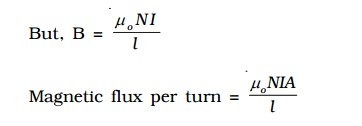Home | | Physics | Self inductance of a long solenoid

# Self inductance of a long solenoid

Let us consider a solenoid of N turns with length l and area of cross section A. It carries a current I.

Self inductance of a long solenoid

Let us consider a solenoid of N turns with length l and area of cross section A. It carries a current I. If B is the magnetic field at any point inside the solenoid, then

Magnetic flux per turn =         B × area of each turnHence, the total magnetic flux (φ) linked with the solenoid is given by the product of flux through each turn and the total number of turns.Study Material, Lecturing Notes, Assignment, Reference, Wiki description explanation, brief detail
Physics : Effects of electric current : Higher Secondary(12 Std) : Self inductance of a long solenoid |